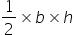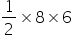Maths-
General
Easy

Question

# The edges of a triangular board are 6 cm, 8 cm and 10 cm. Find the area of triangle.Hint:

## The correct answer is: 24 cm2

### It is given that a = 6 cm , b = 8 cm and c = 10 cmUsing Pythagoras theorem102 = 82 + 62100 = 64 + 36100 = 100  i.e. Pythagoras holds trueHence given triangle is a right angles triangle with Base, b = 8 cm . height, h = 6 cm and hypotenuse = 10 cmArea of a right angle triangle === 24 cm2#### With Turito Foundation.#### Get an Expert Advice From Turito.